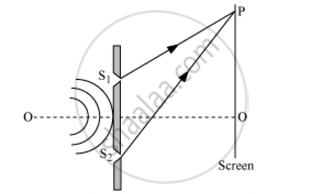Share

# Light Waves Each of Amplitude "a" and Frequency "ω", Emanating from Two Coherent Light Sources Superpose at a Point. - Physics

ConceptPrinciple of Superposition of Waves,

#### Question

Light waves each of amplitude "a" and frequency "ω", emanating from two coherent light sources superpose at a point. If the displacements due to these waves are given by y1 = a cos ωt and y2 = a cos(ωt + ϕ) where ϕ is the phase difference between the two, obtain the expression for the resultant intensity at the point.

#### SolutionLet the displacement of the waves from the sources S1 and S2 at point P on the screen at any time t be given by:

y1 = a cos ωt

and

y2 = a cos (ωt + Φ)

where, Φ is the constant phase difference between the two waves

By the superposition principle, the resultant displacement at point P is given by:

y = y1 + y2

y = a cos ωt + a cos (ωt + Φ)

=2a[cos((omegat+omegat+phi)/2)cos((omegat-omegat-phi)/2)]

y=2acos(omegat+phi/2)cos(phi/2)" ...(i)"

Let 2 acos(phi/2)=A ...(ii)"

Then, equation (i) becomes:

y=Acos(omegat+phi/2)

Now, we have:

A^2=4a^2cos^2(phi/2)" ..(iii)"

The intensity of light is directly proportional to the square of the amplitude of the wave. The intensity of light at point P on the screen is given by:

I=4a^2cos^2(phi/2)" ...(iv)"

Is there an error in this question or solution?

#### Video TutorialsVIEW ALL 

Solution Light Waves Each of Amplitude "a" and Frequency "ω", Emanating from Two Coherent Light Sources Superpose at a Point. Concept: Principle of Superposition of Waves,.
S